computer vision

# useful packages

• https://www.cellpose.org/ - cell segmentation
• Active shape model - Wikipedia
• intro (cootes 2000)
• Model-based methods make use of a prior model of what is expected in the image, and typically attempt to find the best match of the model to the data in a new image
• model
• requires user-specified landmarks $x$ (e.g. points for eyes/nose on a face)
• simplest model - use a typical example as a prototype + compare others using correlation
• invariances: given a set of image coordinates, for all rotations / scales / translations - try them all so that the sum of distances of each shape to the mean is minimized (called Procrustes analysis)
• shape model learns low-dim model of $x$, maybe using $k$ top bases of PCA
• inference
• nearest neighbor: iteratively find transformation + shape model parameters to represent landmarks
• classification: non-trivial to define a goodness of fit measure for the landmarks - something like distance between points and strongest nearby edges
• active shape model - for each landmark, look for nearby groundtruth, adapt PCA values, apply reasonable constraints, then iterate
• improve speed by doing this at large scales before going to more detailed scales
• snakes - active contour models (kass et al. 1988)
• deformable spline - pulled towards object contours while internal forces resist deformation

# what’s in an image?

• vision doesn’t exist in isolation - movement
• three R’s: recognition, reconstruction, reorganization

## fundamentals of image formation

### projections

• image I(x,y) projects scene(X, Y, Z)
• lower case for image, upper case for scene
•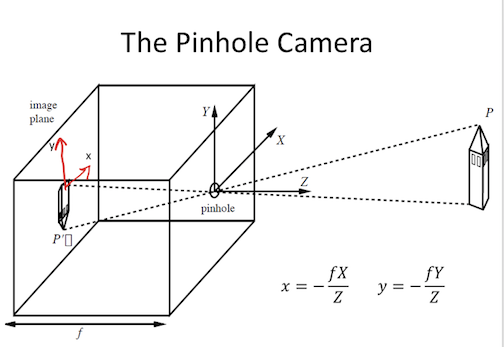• f is a fixed dist. not a function
• box with pinhole=center of projection, which lets light go through
• Z axis points out of box, X and Y aligned w/ image plane (x, y)
• perspective projection - maps 3d points to 2d points through holes
•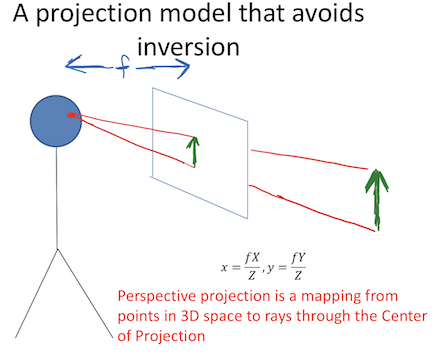• perspective projection works for spherical imaging surface - what’s important is 1-1 mapping between rays and pixels
• natural measure of image size is visual angle
• orthographic projection - appproximation to perspective when object is relatively far
• define constant $s = f/Z_0$
• transform $x = sX, y = sY$

### phenomena from perspective projection

• parallel lines converge to vanishing point (each family has its own vanishing point)
• pf: point on a ray $[x, y, z] = [A_x, A_y, A_z] + \lambda [D_x, D_y, D_z]$
• $x = \frac{fX}{Z} = \frac{f \cdot (A_x+\lambda D_X)}{A_z + \lambda D_z}$
• $\lambda \to \infty \implies \frac{f \cdot \lambda D_x}{\lambda D_z} = \frac{f \cdot D_x}{D_z}$
• $\implies$ vanishing point coordinates are $fD_x / D_z , f D_y / D_z$
• not true when $D_z = 0$
• all vanishing points lie on horizon
• nearer objects are lower in the image
• let ground plane be $Y = -h$ (where h is your height)
• point on ground plane $y = -fh / Z$
• nearer objects look bigger
• foreshortening - objects slanted w.r.t line of sight become smaller w/ scaling factor cos $\sigma$ ~ $\sigma$ is angle between line of sight and the surface normal

• irradiance - how much light (photons) is captured in some time interval
• radiant power / unit area ($W/m^2$)
• radiance - power in given direction / unit area / unit solid angle
• L = directional quantity (measured perpendicular to direction of travel)
• $L = Power / (dA \cos \theta \cdot d\Omega)$ where $d\Omega$ is a solid angle (in steradians)
• irradiance $\propto$ radiance in direction of the camera
• outgoing radiance of a patch has 3 factors
• incoming radiance from light source
• angle between light / camera
• reflectance properties of patch
• 2 special cases
• specular surfaces - outgoing radiance direction obeys angle of incidence
• lambertian surfaces - outgoing radiance same in all directions
• albedo * radiance of light * cos(angle)
• model reflectance as a combination of Lambertian term and specular term
• also illuminated by reflections of other objects (ray tracing / radiosity)
• shape-from-shading (SFS) goes from irradiance $\to$ geometry, reflectances, illumination

## frequencies and colors

• contrast sensitivity depends on frequency + color
• band-pass filtering - use gaussian pyramid
• pyramid blending
• eye
• iris - colored annulus w/ radial muscles
• pupil - hole (aperture) whose size controlled by iris
• retina:• colors are what is reflected
• cones (short = blue, medium = green, long = red)
• metamer - 2 different but indistinguishable spectra
• color spaces
• rgb - easy for devices
• chips tend to be more green
• hsv (hue, saturation, value)
• lab (perceptually uniform color space)
• color constancy - ability to perceive invariant color despite ecological variations
• camera white balancing (when entire photo is too yellow or something)
• manual - choose color-neutral object and normalize
• automatic (AWB)
• grey world - force average color to grey
• white world - force brightest object to white

# image processing

## transformations

• 2 object properties
• pose - position and orientation of object w.r.t. the camera (6 numbers - 3 translation, 3 rotation)
• shape - relative distances of points on the object
• nonrigid objects can change shape
Transform (most general on top) Constraints Invariants 2d params 3d params
Projective = homography (contains perspective proj.) Ax + t, A nonsingular, homogenous coords parallel -> intersecting 8 (-1 for scale) 15 (-1 for scale)
Affine Ax + t, A nonsingular parallelism, midpoints, intersection 6=4+2 12=9+3
Euclidean = Isometry Ax + t, A orthogonal length, angles, area 3=1+2 6=3+3
Orthogonal (rotation when det = 1 / reflection when det = -1) Ax, A orthogonal   1 3
• projective transformation = homography

• homogenous coordinates - use n + 1 coordinates for n-dim space to help us represent points at $\infty$
• $[x, y] \to [x_1, x_2, x_3]$ with $x = x_1/x_3, y=x_2/x_3$
• $[x_1, x_2] = \lambda [x_1, x_2] \quad \forall \lambda \neq 0$ - each points is like a line through origin in n + 1 dimensional space
• standardize - make third coordinate 1 (then top 2 coordinates are euclidean coordinates)
• when third coordinate is 0, other points are infinity
• all 0 disallowed
• Euclidean line $a_1x + a_2y + a_3=0$ $\iff$ homogenous line $a_1 x_1 + a_2x_2 + a_3 x_3 = 0$
• perspective maps parallel lines to lines that intersect
• incidence of points on lines
• when does a point $[x_1, x_2, x_3]$ lie on a line $[a_1, a_2, a_3]$ (homogenous coordinates)
• when $\mathbf{x} \cdot \mathbf{a} = 0$
• cross product gives intersection of any 2 lines
• representing affine transformations: $\begin{bmatrix}X’\Y’\W’\end{bmatrix} = \begin{bmatrix}a_{11} & a_{12} & t_x\ a_{21} & a_{22} & t_y \ 0 & 0 & 1\end{bmatrix}\begin{bmatrix}X\Y\1\end{bmatrix}$
• representing perspective projection: $\begin{bmatrix}1 & 0& 0 & 0\ 0 & 1 & 0 & 0 \ 0 & 0 & 1/f & 0 \end{bmatrix} \begin{bmatrix}X\Y\Z \ 1\end{bmatrix} = \begin{bmatrix}X\Y\Z/f\end{bmatrix} = \begin{bmatrix}fX/Z\fY/Z\1\end{bmatrix}$
• affine transformations
• affine transformations are a a group
• examples
• anisotropic scaling - ex. $\begin{bmatrix}2 & 0 \ 0 & 1 \end{bmatrix}$
• shear
• euclidean transformations = isometries = rigid body transform
•  preserves distances between pairs of points: $\psi(a) - \psi(b) = a-b$
• ex. translation $\psi(a) = a+t$
• composition of 2 isometries is an isometry - they are a group
• orthogonal transformations - preserves inner products $\forall a,b : a \cdot b =a^T A^TA b$
• $\implies A^TA = I \implies A^T = A^{-1}$
• $\implies det(A) = \pm 1$
• 2D
• really only 1 parameter $\theta$ (also for the +t)
• $A = \begin{bmatrix}cos \theta & - sin \theta \ sin \theta & cos \theta \end{bmatrix}$ - rotation, det = +1
• $A = \begin{bmatrix}cos \theta & sin \theta \ sin \theta & - cos \theta \end{bmatrix}$ - reflection, det = -1
• 3D
• really only 3 parameters
• ex. $A = \begin{bmatrix}cos \theta & - sin \theta & 0 \ sin \theta & cos \theta & 0 \ 0 & 0 & 1\end{bmatrix}$ - rotation, det rotate about z-axis (like before)
• rotation - orthogonal transformations with det = +1
• 2D: $\begin{bmatrix}cos \theta & - sin \theta \ sin \theta & cos \theta \end{bmatrix}$
• 3D: $\begin{bmatrix}cos \theta & - sin \theta & 0 \ sin \theta & cos \theta & 0 \ 0 & 0 & 1\end{bmatrix}$ (rotate around z-axis)
• lots of ways to specify angles
• axis plus amount of rotation - we will use this
• euler angles
• quaternions (generalize complex numbers)
• Roderigues formula - converts: $R = e^{\phi \hat{s}} = I + sin [\phi] : \hat{s} + (1 - cos \phi) \hat{s}^2$
•  $s$ is a unit vector along $w$ and $\phi= w t$ is total amount of rotation
• rotation matrix
• can replace cross product with matrix multiplication with a skew symmetric $(B^T = -B)$ matrix:
• $\begin{bmatrix} t_1 \ t_2 \ t_3\end{bmatrix}$ ^ $\begin{bmatrix} x_1 \ x_2 \ x_3 \end{bmatrix} = \begin{bmatrix} t_2 x_3 - t_3 x_2 \ t_3 x_1 - t_1 x_3 \ t_1 x_2 - t_2 x_1\end{bmatrix}$
• $\hat{t} = [t_\times] = \begin{bmatrix} 0 & -t_3 & t_2 \ t_3 & 0 & -t_1 \ -t_2 & t_1 & 0\end{bmatrix}$
• proof
• $\dot{q(t)} = \hat{w} q(t)$
• $\implies q(t) = e^{\hat{w}t}q(0)$
• where $e^{\hat{w}t} = I + \hat{w} t + (\hat{w}t)^2 / w! + …$
• can rewrite in terms above

## image preprocessing

• image is a function from $R^2 \to R$
• f(x,y) = reflectance(x,y) * illumination(x,y)
• image histograms - treat each pixel independently
• better to look at CDF
• use CDF as mapping to normalize a histogram
• histogram matching - try to get histograms of all pixels to be same
• need to map high dynamic range (HDR) to 0-255 by ignoring lots of values
• do this with long exposure
• point processing does this transformation independent of position x, y
• can enhance photos with different functions
• negative - inverts
• log - can bring out details if range was too large
• contrast stretching - stretch the value within a certain range (high contrast has wide histogram of values)
• sampling
• sample and write function’s value at many points
• reconstruction - make samples back into continuous function
• ex. audio -> digital -> audio
• undersampling loses information
• aliasing - signals traveling in disguise as other frequencies
•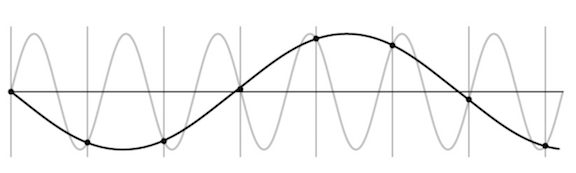•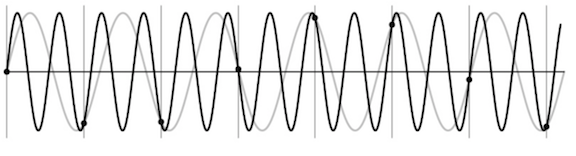• antialiasing
• can sample more often
• make signal less wiggly by removing high frequencies first
• filtering
• lowpass filter - removes high frequencies
• linear filtering - can be modeled by convolution
• cross correlation - what cnns do, dot product between kernel and neighborhood
• sobel filter is edge detector
• gaussian filter - blur, better than just box blur
• rule of thumb - set filter width to about 6 $\sigma$
• removes high-frequency components
• convolution - cross-correlation where filter is flipped horizontally and vertically
• commutative and associative
• convolution theorem: $F[g*h] = F[g]F[h]$ where F is Fourier, * is convolution
• convolution in spatial domain = multiplication in frequency domain
• resizing
• Gaussian (lowpass) then subsample to avoid aliasing
• image pyramid - called pyramid because you can subsample after you blur each time
• whole pyramid isn’t much bigger than original image
• collapse pyramid - keep upsampling and adding
• good for template matching, search over translations
• sharpening - add back the high frequencies you remove by blurring (laplacian pyramid):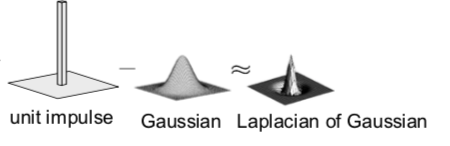## edges + templates

• edge - place of rapid change in the image intensity function
• solns

• smooth first, then take gradient
• gradient first then smooth gives same results (linear operations are interchangeable)
• derivative theorem of convolution - differentiation can also be though of as convolution

• can convolve with deriv of gaussian
• can give orientation of edges
• tradeoff between smoothing (denoising) and good edge localization (not getting blurry edges)
• image gradient looks like edges
• canny edge detector

1. filter image w/ deriv of Gaussian
2. find magnitude + orientation of gradient
3. non-maximum suppression - does thinning, check if pixel is local maxima
• anything that’s not a local maximum is set to 0
• on line direction, require a weighted average to interpolate between points (bilinear interpolation = average on edges, then average those points)
4. hysteresis thresholding - high threshold to start edge curves then low threshold to continue them
• Scale-space and edge detection using anisotropic diffusion (perona & malik 1990)
• introduces anisotropic diffusion (see wiki page) - removes image noise without removing content
• produces series of images, similar to repeatedly convolving with Gaussian
• filter review
• smoothing
• no negative values
• should sum to 1 (constant response on constant)
• derivative
• must have negative values
• should sum to 0 (0 response on constant)
• intuitive to have positive sum to +1, negative sum to -1
• matching with filters (increasing accuracy, but slower)
• ex. zero-mean filter subtract mean of patch from patch (otherwise might just match brightest regions)
• ex. SSD - L2 norm with filter
• doesn’t deal well with intensities
• ex. normalized cross-correlation
• recognition
• instance - “find me this particular chair”
• simple template matching can work
• category - “find me all chairs”

## texture

• texture - non-countable stuff
• related to material, but different
• texture analysis - compare 2 things, see if they’re made of same stuff
• pioneered by bela julesz
• random dot stereograms - eyes can find subtle differences in randomness if fed to different eyes
• human vision sensitive to some difference types, but not others
• easy to classify textures based on v1 gabor-like features
• can make histogram of filter response histograms - convolve filter with image and then treat each pixel independently
• heeger & bergen siggraph 95 - given texture, want to make more of that texture
• match histograms of noise with each of your filter responses
• combine them back together to make an image
• repeat this iteratively
• simoncelli + portilla 98 - also match 2nd order statistics (match filters pairwise)
• much harder, but works better
• texton histogram matching - classify images
• use “computational version of textons” - histograms of joint responses
• like bag of words but with “visual words”
• won’t get patches with exact same distribution, so need to extract good “words”
• define words as k-means of features from 10x10 patches
• features could be raw pixels
• gabor representation ~10 dimensional vector
• SIFT features: histogram set of oriented filters within each box of grid
• HOG features
• usually cluster over a bunch of images
• invariance - ex. blur signal
• each image patch -> a k-means cluster so image -> histogram
• then just do nearest neighbor on this histogram (chi-squared test is good metric)
• object recognition is really texture recognition
• all methods follow these steps
• compute low-level features
• aggregate features - k-means, pool histogram
• use as visual representation
• why these filters - sparse coding (data driven find filters)

## optical flow

• simplifying assumption - world doesn’t move, camera moves
• lets us always use projection relationship $x, y = -Xf/Z, -Yf/Z$
• optical flow - movement in the image plane
• square of points moves out as you get closer
• as you move towards something, the center doesn’t change
• things closer to you change faster
• if you move left / right points move in opposite direction
• rotations also appear to move opposite to way you turn your head
• equations: relate optical flow in image to world coords
• optical flow at $(u, v) = (\Delta x / \Delta t, \Delta y/ \Delta t)$ in time $\Delta t$
• function in image space (produces vector field)
• $\begin{bmatrix} \dot{X}\ \dot{Y} \ \dot{Z} \end{bmatrix} = -t -\omega \times \begin{bmatrix} X \ Y \ Z\end{bmatrix} \implies \begin{bmatrix} \dot{x}\ \dot{y}\end{bmatrix}= \frac{1}{Z} \begin{bmatrix} -1 & 0 & x\ 0 & 1 & y\end{bmatrix} \begin{bmatrix} t_x \ t_y \ t_z \end{bmatrix}+ \begin{bmatrix} xy & -(1+x^2) & y \ 1+y^2 & -xy & -x\end{bmatrix}\begin{bmatrix} \omega_x \ \omega_y \ \omega_z\end{bmatrix}$
• decomposed into translation component + rotation component
• $t_z / Z$ is time to impact for a point
• translational component of flow fields is more important - tells us $Z(x, y)$ and translation $t$
• we can compute the time to contact
• this is a key to what is used in video compression

# cogsci / neuro

## psychophysics

• julesz search experiment
• “pop-out” effect of certain shapes (e.g. triangles but not others)
• axiom 1: human vision has 2 modes
• preattentive vision - parallel, instantaneous (~100-200 ms)
• large visual field, no scrutiny
• surprisingly large amount of what we do
• ex. sensitive to size/width, orientation changes
• attentive vision - serial search with small focal attention in 50 ms steps
• axiom 2: textons are the fundamental elements in preattentive vision
• texton is invariant in preattentive vision
• ex. elongated blobs (rectangles, ellipses, line segments w/ orientation/width/length)
• ex. terminators - ends of line segments
• crossing of line segments
• julesz conjecture (not quite true) - textures can’t be spontaneously discriminated if they have same first-order + second-order statistics (ex. density)
• humans can saccade to correct place in object detection really fast (150 ms - Kirchner & Thorpe, 2006)
• still in preattentive regime
• can also do object detection after seeing image for only 40 ms

## neurophysiology

• on-center off-surround - looks like Laplacian of a Gaussian
• horizontal cell “like convolution”
• LGN does quick processing
• hubel & wiesel - single-cell recording from visual cortex in v1
• 3 v1 cell classes
• simple cells - sensitive to oriented lines
• oriented Gaussian derivatives
• some were end-stopped
• complex cells - simple cells with some shift invariance (oriented lines but with shifts)
• could do this with maxpool on simple cells
• hypercomplex cells (less common) - complex cell, but only lines of certain length
• hypercolumn - cells of different orientations, scales grouped close together for a location

## perceptual organization

• max werthermian - we perceive things not numbers
• principles: grouping, element connectedness
• figure-ground organization: surroundedness, size, orientation, contrast, symmetry, convexity
• gestalt - we see based on context

# correspondence + applications (steropsis, optical flow, sfm)

## binocular steropsis

• stereopsis - perception of depth
• disparity - difference in image between eyes
• this signals depth (0 disparity at infinity)
• measured in pixels (in retina plane) or angle in degrees
• sign doesn’t really matter
• active stereopsis - one projector and one camera vs passive (ex. eyes)
• active uses more energy
• ex. kinect - measure / triangulate
• worse outside
• ex. lidar - time of light - see how long it takes for light to bounce back
• 3 types of 2-camera configurations: single point, parallel axes, general case

### single point of fixation (common in eyes)

• fixation point has 0 disparity
• humans do this to put things in the fovea
• use coordinates of cyclopean eye
• vergence movement - look at close / far point on same line
• change angle of convergence (goes to 0 at $\infty$)
• disparity = $2 \delta \theta = b \cdot \delta Z / Z^2$ where b - distance between eyes, $\delta Z$ - change in depth, Z - depth
•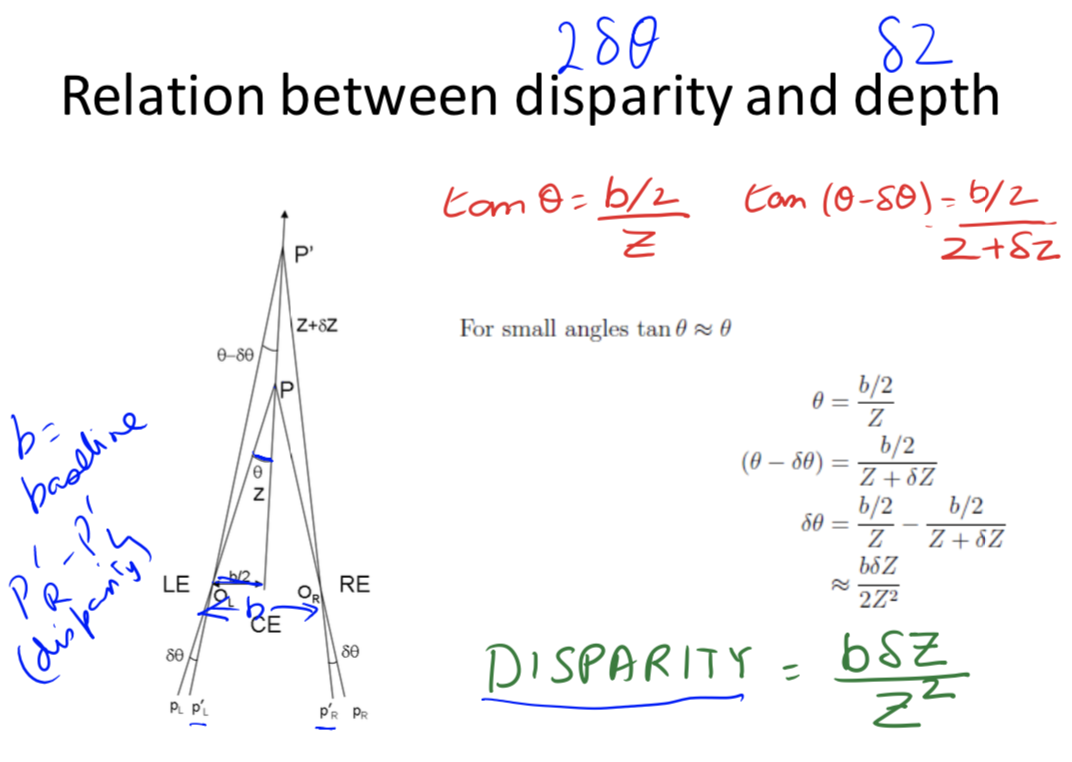• b - distance between eyes, $\delta$ - change in depth, Z - depth
• version movement - change direction of gaze
• forms Vieth-Muller circle - points lie on same circle with eyes
• cyclopean eye isn’t on circle, but close enough
• disparity of P’ = $\alpha - \beta = 0$ on Vieth-Muller circle
•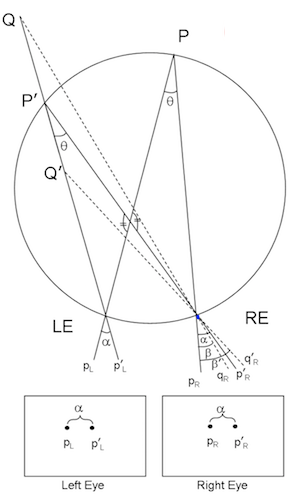### optical axes parallel (common in robots)

•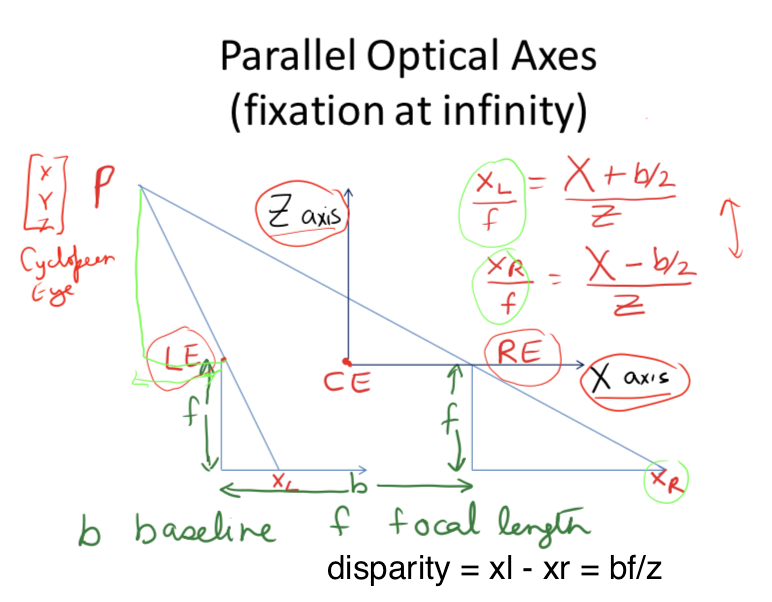• disparity $d = x_l - x_r = bf/Z$
•  error $\delta Z = \frac{Z^2 \delta d }{bf}$
• parallax - effect where near objects move when you move but far don’t

### general case (ex. reconstruct from lots of photos)

• given n point correspondences, estimate rotation matrix R, translation t, and depths at the n points

• more difficult - don’t know coordinates / rotations of different cameras
• epipolar plane - contains cameras, point of fixation

• different epipolar planes, but all contain line between cameras
• $\vec{c_1 c_2}$ is on all epipolar planes
• each image plane has corresponding epipolar line - intersection of epipolar plane with image plane
• epipole - intersection of $\vec{c_1 c_2}$ and image plane
•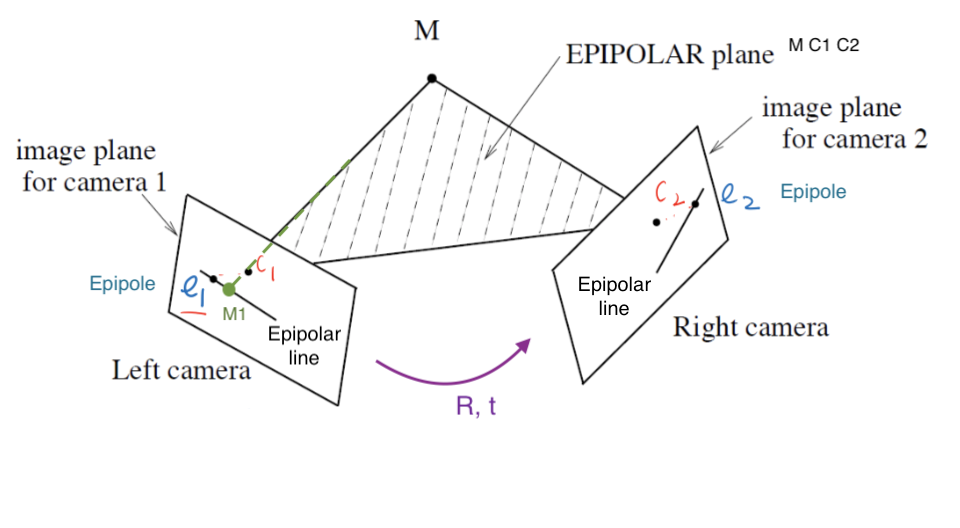• structure from motion problem: given n corresponding projections $(x_i, y_i)$ in both cameras, find $(X_i, Y_i, Z_i)$ by estimating R, t: Longuet-Higgins 8-point algorithm - overall minimizing re-projection error (basically minimizes least squares = bundle adjustment)

• find $n (\geq 8)$ corresponding points
• estimate essential matrix $E = \hat{T} R$ (converts between points in normalized image coords - origin at optical center)
• fundamental matrix F corresponds between points in pixel coordinates (more degrees of freedom, coordinates not calibrated )
• essential matrix constraint: $x_1, x_2$ homogoneous coordinates of $M_1, M_2 \implies x_2^T \hat{T} R x_1 = 0$
• 6 or 5 dof; 3 dof for rotation, 3 dof for translation. up to a scale, so 1 dof is removed
• $t = c_2 - c_1, x_2$ in second camera coords, $Rx_1$ moves 1st camera coords to second camera coords
• need at least 8 pairs of corresponding points to estimate E (since E has 8 entries up to scale)
• if they’re all coplanar, etc doesn’t always work (need them to be independent)
• extract (R, t)
• triangulation

## solving for stereo correspondence

• stereo correspondence = stereo matching: given point in one image, find corresponding point in 2nd image
• basic stereo matching algorithm
• stereo image rectification - transform images so that image planes are parallel
• now, epipolar lines are horizontal scan lines
• do this by using a few points to estimate R, t
• for each pixel in 1st image
• find corresponding epipolar line in 2nd image
• correspondence search: search this line and pick best match
• simple ex. parallel optical axes = assume cameras at same height, same focal lengths $\implies$ epipolar lines are horizontal scan lines
•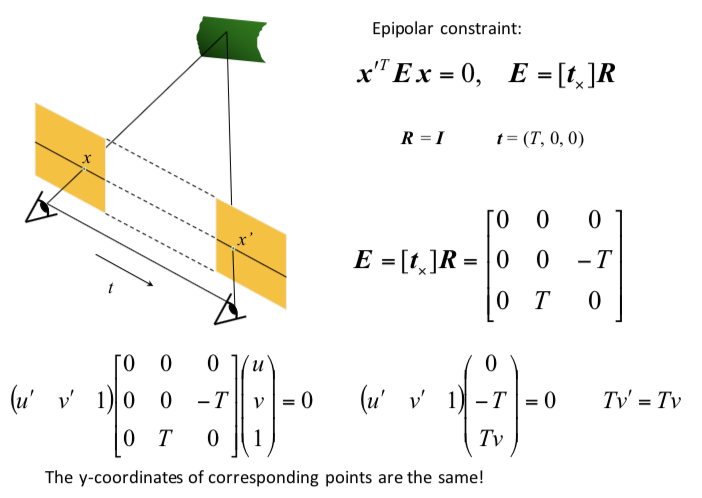• correspondence search algorithms (simplest to most complex)
• assume photo consistency - same points in space will give same brightness of pixels
• take a window and use metric
• larger window smoother, less detail
• metrics
• minimize L2 norm (SSD)
• maximize dot product (NCC - normalized cross correlation) - this works a little better because calibration issues could be different
• failures
• textureless surfaces
• occlusions - have to extrapolate the disparity
• half-occlusion - can’t see from one eye
• full-occlusion - can’t see from either eye
• repetitions
• non-lambertian surfacies, specularities - mirror has different brightness from different angles

### optical flow II

• related to stereo disparity except moving one camera over time
• aperture problem - looking through certain hole can change perception (ex. see movement in wrong directions)
• measure correspondence over time
• for point (x, y, t), optical flow is (u,v) = $(\Delta x / \Delta t, \Delta y / \Delta t)$
• optical flow constraint equation: $I_x u + I_y v + I_t = 0$
• assume everything is Lambertian - brightness of any given point will stay the same
• also add brightness constancy assumption - assume brightness of given point remains constant over short period $I(x_1, y_1, t_1) = I(x_1 + \Delta x, y_1 + \Delta x, t_1 + \Delta t)$
• here, $I_x = \partial I / \partial x$

• local constancy of optical flow - assume u and v are same for n points in neighborhood of a pixel
• rewrite for n points(left matrix is A): $\begin{bmatrix} I_x^1 & I_y^1\ I_x^2 & I_y^2\ \vdots & \vdots \ I_x^n & I_y^n\ \end{bmatrix}\begin{bmatrix} u \ v\end{bmatrix} = - \begin{bmatrix} I_t^1\ I_t^2\ \vdots \ I_t^n\\end{bmatrix}$
• then solve with least squares $\begin{bmatrix} u \ v\end{bmatrix}=-(A^TA^{-1} A^Tb)$
• second moment matrix $A^TA$ - need this to be high enough rank

## general correspondence + interest points

• more general correspondence - matching points, patches, edges, or regions across images (not in the same basic image)
• most important problem - used in steropsis, optical flow, structure from motion
• 2 ways of finding correspondences
• align and search - not really used
• keypoint matching - find keypoint that matches and use everything else
• 3 steps to kepoint matching: detection, description, matching

### detection - identify key points

• find ‘corners’ with Harris corner detector
• shift small window and look for large intensity change in multiple directions
• edge - only changes in one direction
• compare auto-correlation of window (L2 norm of pixelwise differences)
• very slow naively - instead look at gradient (Taylor series expansion - second moment matrix M)
• if gradient isn’t flat, then it’s a corner
• look at eigenvalues of M
• eigenvalues tell you about magnitude of change in different directions
• if same, then circular otherwise elliptical
• corner - 2 large eigenvalues, similar values
• edge - 1 eigenvalue larger than other
• simple way to compute this: $det(M) - \alpha : trace(M)^2$
• apply max filter to get rid of noise
• adaptive - want to distribute points across image
• invariance properties
• ignores affine intensity change (only uses derivs)
• ignores translation/rotation
• does not ignore scale (can fix this by considering multiple scales and taking max)

### description - extract vector feature for each key point

• lots of ways - ex. SIFT, image patches wrt gradient
• simpler: MOPS
• take point (x, y), scale (s), and orientation from gradients
• take downsampled rectangle around this point in proper orientation
• invariant to things like shape / lighting changes

### matching - determine correspondence between 2 views

• not all key points will match - only match above some threshold
• ex. criteria: symmetry - only use if a is b’s nearest neighbor and b is a’s nearest neighbor
• better: David Lowe trick - how much better is 1-NN than 2-NN (e.g. threshold on 1-NN / 2-NN)
• problem: outliers will destroy fit
• RANSAC algorithm (random sample consensus) - vote for best transformation
• repeat this lots of times, pick the match that had the most inliers
• select n feature pairs at random (n = minimum needed to compute transformation - 4 for homography, 8 for rotation/translation)
• compute transformation T (exact for homography, or use 8-point algorithm)
• count inliers (how many things agree with this match)
• 8-point algorithm / homography check
• $x^TEx < \epsilon$ for 8-point algorithm or $x^THx < \epsilon$ for homography
• finally, could recompute least squares H or F on all inliers

## correspondence for sfm / instance retrieval

• sfm (structure for motion) - given many images, simultaneously do 2 things

• calibration - find camera parameters
• triangulation - find 3d points from 2d points
• structure for motion system (ex. photo tourism 2006 paper)
• camera calibration: determine camera parameters from known 3d points
• parameters
1. internal parameters - ex. focal length, optical center, aspect ratio
2. external parameters - where is the camera
• only makes sense for multiple cameras
• approach 1 - solve for projection matrix (which contains all parameters)
• requires knowing the correspondences between image and 3d points (can use calibration object)
• least squares to find points from 3x4 projection matrix which projects (X, Y, Z, 1) -> (x, y, 1)
• approach 2 - solve for parameters
• translation T, rotation R, focal length f, principle point (xc, yc), pixel size (sx, sy)
• can’t use homography because there are translations with changing depth
• sometimes camera will just list focal length
• decompose projection matrix into a matrix dependent on these things
• nonlinear optimization
• triangulation - predict 3d points $(X_i, Y_i, Z_i)$ given pixels in multiple cameras $(x_i, y_i)$ and camera parameters $R, t$
•  minimize reprojection error (bundle adjustment): $\sum_i \sum_j \underbrace{w_{ij}}_{\text{indicator var}}\cdot \underbrace{P(x_i, R_j, t_j)}{\text{pred. im location}} - \underbrace{\begin{bmatrix} u{i, j}\v_{i, j}\end{bmatrix}}_{\text{observed m location}} ^2$
• solve for matrix that projects points into 3d coords
• initial pair should have lots of matches, big baseline (shouldn’t just be a homography)
• solve with essential matrix
• then iteratively add cameras and recompute
• good idea: ignore lots of data since data is cheap in computer vision
• search for similar images - want to establish correspondence despite lots of changes
• see how many keypoint matches we get
• search with inverted file index
• ex. visual words - cluster the feature descriptors and use these as keys to a dictionary
• inverted file indexing
• should be sparse
• spatial verification - don’t just use visual words, use structure of where the words are
• want visual words to give similar transformation - RANSAC with some constraint

# deep learning

## cnns

• object recognition - visual similarity via labels
• classification
• linear boundary -> nearest neighbors
• neural nets
• don’t need feature extraction step
• high capacity (like nearest neighbors)
• still very fast test time
• good at high dimensional noisy inputs (vision + audio)
• pooling - kind of robust to exact locations
• a lot like blurring / downsampling
• everyone now uses maxpooling
• history: lenet 1998
• neocognitron (fukushima 1980) - unsupervised
• convolutional neural nets (lecun et al) - supervised
• alexnet 2012
• used norm layers (still common?)
• resnet 2015
• 152 layers
• 3x3s with skip layers
• like nonparametric - number of params is close to number of data points
• network representations learn a lot
• zeiler-fergus - supercategories are learned to be separated, even though only given single class lavels
• nearest neighbors in embedding spaces learn things like pose
• can be used for transfer learning
• fancy architectures - not just a classifier
• siamese nets
• ex. want to compare two things (ex. surveillance) - check if 2 people are the same (even w/ sunglasses)
• ex. connect pictures to amazon pictures
• embed things and make loss function distance between real pics and amazon pics + make different things farther up to some margin
• ex. searching across categories
• multi-modal
• ex. could look at repr. between image and caption
• semi-supervised
• context as supervision - from word predict neighbors
• predict neighboring patch from 8 patches in image
• many tasks / many losses at once - everything will get better at once
• differentiable programming - any nets that form a DAG
• if there are cycles (RNN), unroll it
• fully convolutional
• works on different sizes
• this lets us have things per pixel, not per image (ex. semantic segmentation, colorization)
• usually use skip connections

## image segmentation

• consistency - 2 segmentations consistent when they can be explained by same segmentation tree
• percept tree - describe what’s in an image using a tree
• evaluation - how to correspond boundaries?
• min-cost assignment on bipartite graph=bigraph - connections only between groundtruth, signal: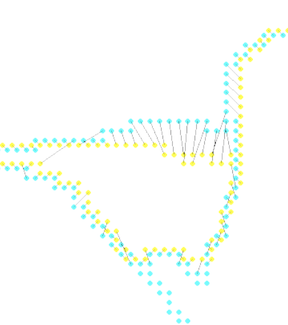• ex. for each pixel predict if it’s on a boundary by looking at window around it
• proximity cue
• look for sharp change in the property
• region cue - patch similarity
• proximity
• graph partitioning
• learn cue combination by fitting linear combination of cues and outputting whether 2 pixels are in same segmentation
• graph partitioning approach: generate affinity graph from local cues above (with lots of neighbors)
• normalized cuts - partition so within-group similarity is large and between-group similarity is small
• deep semantic segmentation - fully convolutional
• upsampling
• unpooling - can fill all, always put at top-left
• max-unpooling - use positions from pooling layer
• learnable upsampling = deconvolution = upconvolution = fractionally strided convolution = backward strided convolution - transpose the convolution

## classification + localization

• goal: coords (x, y, w, h) for each object + class
• simple: sliding window and use classifier each time - computationally expensive!
• region proposals - find blobby image regions likely to contain objects and run (fast)
• R-CNN - run each region of interest, warped to some size, through CNN
• Fast R-CNN - get ROIs from last conv layer, so everything is faster / no warping
• to maintain size, fix number of bins instead of filter sizes (then these bins are adaptively sized) - spatial pyramid pooling layer
• Faster R-CNN - use region proposal network within network to do region proposals as well
• train with 4 losses for all things needed
• region proposal network uses multi-scale anchors and predicts relative to convolution
• instance segmentation
• mask-rcnn - keypoint detection then segmentation

## learning detection

• countour detection - predict contour after every conv (at different scales) then interpolate up to get one final output (ICCV 2015)
• deep supervision helps to aggregate multiscale info
• semantic segmentation - sliding window
• classification + localization
• need to output a bounding box + classify what’s in the box
• bounding box: regression problem to output box
• use locations of features
• feature map
• location of a feature in a feature map is where it is in the image (with finer localization info accross channels)
• response of a feature - what it is

### modeling figure-ground

• figure is closer, ground background - affects perception
• figure/ground datasets
• local cues
• edge features: shapemes - prototypical local shapes
• junction features: line labelling - contour directions with convex/concave images
• lots of principles
• surroundedness, size, orientation, constrast, symmetry, convexity, parallelism, lower region, meaningfulness, occlusion, cast shadows, shading
• global cues
• want consistency with CRF
• spectral graph segmentation
• embedding approach - satisfy pairwise affinities

## single-view 3d construction

• useful for planning, depth, etc.
• different levels of output (increasing complexity)
• image depth map
• scene layout - predict simple shapes of things
• volumetric 3d - predict 3d binary voxels for which voxels are occupied
• could approximate these with CAD models, deformable shape models
• need to use priors of the world
• (explicit) single-view modeling - assume a model and fit it
• many classes are very difficult to model explicitly
• ex. use dominant edges in a few directions to calculate vanishing points and then align things
• (implicit) single-view prediction - learn model of world data-driven
• collect data + labels (ex. sensors)
• train + predict
• supervision from annotation can be wrong